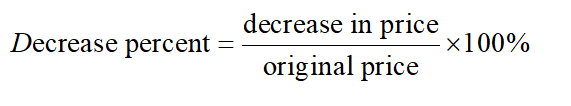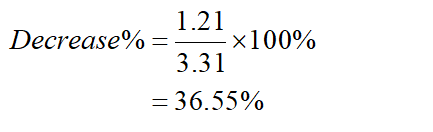# If the price of gas was on average \$2.10 per gallon, and this was \$1.21 cheaper than a year before, what is the percent of decrease in price? (Round to the nearest percent.)

Question
1 views

If the price of gas was on average \$2.10 per gallon, and this was \$1.21 cheaper than a year before, what is the percent of decrease in price? (Round to the nearest percent.)

check_circle

Step 1

Decrease in price is given by,

Step 2

Determine the original price,

original price= current price+ change in prices.

=\$2.10+1.21

=\$3.31

Step 3

Here, decrease in price= 1.21

determine the decrease percent....

### Want to see the full answer?

See Solution

#### Want to see this answer and more?

Solutions are written by subject experts who are available 24/7. Questions are typically answered within 1 hour.*

See Solution
*Response times may vary by subject and question.
Tagged in

### Math